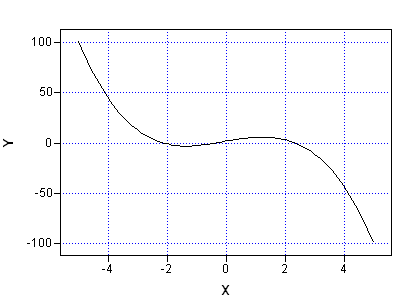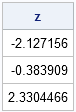Finding the root (or zero) of a function is an important computational task because it enables you to solve nonlinear equations. I have previously blogged about using Newton's method to find a root for a function of several variables. I have also blogged about how to use the bisection method to find the zeros of a univariate function.

As of SAS/IML 12.1, there is an easy way to find the roots of function of one variable. The FROOT function solves for a root on a finite interval by using Brent’s method, which uses a combination of bisection, linear interpolation, and quadratic interpolation to converge to a root. Unlike Newton's method, Brent's method does not require that you specify a derivative for the function. All you need to provide is a module that evaluates the function, f, and an interval [a, b] such that f(a) and f(b) have different signs.

If there is a root in the interval, Brent's method is guaranteed to find it.

As an example, the following SAS/IML module defines a function that I investigated in a previous blog post:

```proc iml; start Func(x); return( exp(-x##2) - x##3 + 5#x +1 ); finish;```

The image at the beginning of this article shows the graph of the function on the interval [–5, 5]. The function has three roots. You can use the graph to estimate intervals on which the function contains a root. The FROOT function enables you to find multiple zeros with a single call, as follows:

```intervals = {-4 -1.5, /* 1st interval [-4, -1.5] */ -1.5 1 , /* 2nd interval [-1.5 1] */ 1 4 }; /* 3rd interval [1, 4] */ z = froot("Func", intervals); print z;```The vector z contains three elements. The first element is the root in the first specified interval, the second element is the root in the second interval, and so forth. If you specify an interval on which the function does not have a root, then the FROOT function returns a missing value.

That's all there is to it. So next time you need to solve a nonlinear equation of one variable, remember that the FROOT function in the SAS/IML language makes the task simple.

ShareDistinguished Researcher in Computational Statistics

Rick Wicklin, PhD, is a distinguished researcher in computational statistics at SAS and is a principal developer of SAS/IML software. His areas of expertise include computational statistics, simulation, statistical graphics, and modern methods in statistical data analysis. Rick is author of the books Statistical Programming with SAS/IML Software and Simulating Data with SAS.

1.Hi,

Just curious, looks like you have to specify each interval, and have to know that each interval has 0 or 1 roots in it?

What happens if there are multiple rooots in your inteval, i.e. intervals={-4 4} would it error or return the first root found or return all three?

Maybe it's a silly question.... I haven't used IML much, but still find your IML posts interesting!

Thx

•It is an excellent question. The answer is that FROOT will find one root. In the example above, if I specify the interval [-4,4], FROOT will return the zero at 2.33. If you look back at my article on the bisection method, you will see that the same thing (finding one root) occurs with bisection. Newton's method would also find one root; which one it finds depends on the initial guess.

2.Rick Aultman on

Question. How can one integrate out on y for function G(x,y) and then find the roots of F(x) - k (where k is a constant) over a range of intervals?

3.Amandeep.singh on

F(z)= 2×cube of z + 4× square of z + 3z +1 . What is the number of roots of polynomial

•The Fundamental Theorem of Algebra tells you that a polynomial of degree n has exactly n complex roots. There is no simple analytical method to determine the number of real roots for an arbitrary polynomial, but a cubic always has 1 or 3 roots (counted with multiplicity). With calculus, you can prove that your cubic polynomial has exactly one real root.